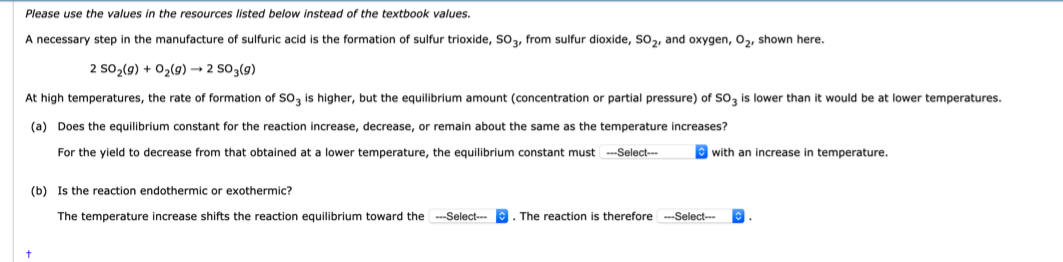# Please use the values in the resources listed below instead of the textbook values. A necessary step in the manufacture of sulfuric acid is the formation of sulfur trioxide, SO3, from sulfur dioxide, SO2, and oxygen, 02, shown here. 2 S02(g) + 02(g) 2 503(g) At high temperatures, the rate of formation of SO3 is higher, but the equilibrium amount (concentration or partial pressure) of SO3 is lower than it would be at lower temperatures. (a) Does the equilibrium constant for the reaction increase, decrease, or remain about the same as the temperature increases? For the yield to decrease from that obtained at a lower temperature, the equilibrium constant must _____with an increase in temperature. (b) Is the reaction endothermic or exothermic? The temperature increase shifts the reaction equilibrium toward the _____. The reaction is therefore ______